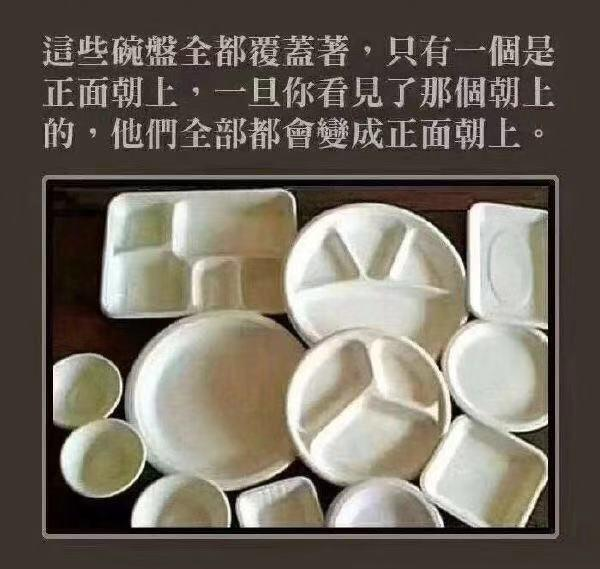# 到底是朝上还是朝下1. 为什么这些碗盘有时候看上去都朝下，有时候又都朝上？
2. 为什么多数人开始看到的是都朝下，而不是都朝上？
3. 为什么一个朝上的碗就能把所有的碗盘都翻过来，而且一旦看到朝上的碗，就再也看不到（或很难再看到）朝下的碗盘了？

# 如何写好竞赛论文摘要

• 摘要有多重要

摘要是竞赛论文中最重要的一部分。

• 竞赛摘要最常见的缺陷

笼统，不具体，信息量不足，简单复述题目要求完成的任务。

• 为什么不能模仿常规摘要写竞赛摘要

常规摘要描述的对象是论文，竞赛摘要描述的对象是模型和方法。常规摘要只需要说明这篇论文做了什么事情，而竞赛摘要要讲清楚是如何做的。

• 竞赛摘要到底要写什么

要写关于模型和方法的实质内容，展示细节，回答题目要求，体现亮点。

• 练习建议

仔细阅读竞赛优秀论文的正文，不看摘要，自己写摘要，再与摘要的原文对比优劣。有条件的话，请经验丰富的老师点评。

# PWWs-01-10

Trisection of a Line Segment1 2 3 4 5 6 7 8 9 10 11 12 13 14 15 16 17 18 19 20 21 22 23 24 25 26 27 28 29 30 31 32 33 34 35 36 37 38 39 40 41 42 43 44 45 46 47 48 49 50 51 52 53 54 55 56 57 58 59 60 61 62 63 \documentclass[tikz, border=2pt]{standalone} \usetikzlibrary{calc} \usetikzlibrary{math} \begin{document} \begin{tikzpicture}[line width=1pt] \tikzmath{ \r = 2; } \def\template#1; { \draw #1 circle [radius=\r]; \draw #1 ++(\r, 0) circle [radius=\r]; \draw #1 -- ++(\r, 0); } \coordinate (O) at (0, 0); \template{(O)}; \node[left] (A) at (O) {$A$}; \node[right] (B) at ($(O) + (\r, 0)$) {$B$}; \coordinate (O1) at (3.5*\r, 0); \template{(O1)}; \coordinate (C1) at ($(O1) + (60:\r)$); \node[above] () at (C1) {$C$}; \coordinate (D1) at ($(O1) + (-120:\r)$); \node[below] () at (D1) {$D$}; \draw (C1) -- (D1); \coordinate (O2) at (0, -3*\r); \template{(O2)}; \coordinate (B2) at ($(O2) + (\r, 0)$); \coordinate (C2) at ($(O2) + (60:\r)$); \coordinate (D2) at ($(O2) + (-120:\r)$); \coordinate (E2) at ($(O2) + (-60:0.5*\r)$); \coordinate (G2) at ($(O2) + (-60:\r)$); \draw (C2) -- (D2); \draw (D2) -- (B2); \draw (O2) -- (G2); \node[right=2mm] () at (E2) {$E$}; \coordinate (O3) at (3.5*\r, -3*\r); \template{(O3)}; \coordinate (B3) at ($(O3) + (\r, 0)$); \coordinate (C3) at ($(O3) + (60:\r)$); \coordinate (D3) at ($(O3) + (-120:\r)$); \coordinate (E3) at ($(O3) + (-60:0.5*\r)$); \coordinate (G3) at ($(O3) + (-60:\r)$); \draw (C3) -- (D3); \draw (D3) -- (B3); \draw (O3) -- (G3); \draw (E3) -- (C3); \draw[dashed] (B3) -- (C3); \node[left] () at (O3) {$A$}; \node[right] () at (B3) {$B$}; \node[above] () at (C3) {$C$}; \node[below] () at (D3) {$D$}; \node[right=2mm] () at (E3) {$E$}; \node[below right] (F3) at ($(O3) + (0.34*\r, 0)$) {$F$}; \node[below=2cm, align=center] () at ($(O2) + (2.25*\r, 0)$) { $\displaystyle\overline{AF} = \frac{1}{3}\cdot\overline{AB}$ } \end{tikzpicture} \end{document} 

# SIAM Awards

COMAP 近日修改了 MCM/ICM竞赛指南 ，SIAM Award（工业与应用数学学会特别奖）仅在MCM竞赛的A题和B题中各评选一个队。